Octave/Matlab - 2D Graph                                                              Home : www.sharetechnote.com

In this page, I would post a quick reference for Matlab and Octave. (Octave is a GNU program which is designed to provide a free tool that work like Matlab. I don't think it has 100% compatability between Octave and Matlab, but I noticed that most of basic commands are compatible. I would try to list those commands that can work both with Matlab and Octave). All the sample code listed here, I tried with Octave, not with Matlab.

There are huge number of functions which has not been explained here, but I would try to list those functions which are most commonly used in most of matlab sample script you can get. My purpose is to provide you the set of basic commands with examples so that you can at least read the most of sample script you can get from here and there (e.g, internet) without overwhelming you. If you get familiar with these minimal set of functionality, you would get some 'feeling' about the tool and then you would make sense out of the official document from Mathworks or GNU Octave which explains all the functions but not so many examples.

I haven't completed 'what I think is the minimum set' yet and I hope I can complete within a couple of weeks. Stay tuned !!!

2D Graph

< Plot only one data set (y axis data only) >

Case 1 : plot(y); // specify y (independent variable) only

Ex)

 Input x = -2*pi:1/10*pi:2*pi; y=sin(x); plot(y); Output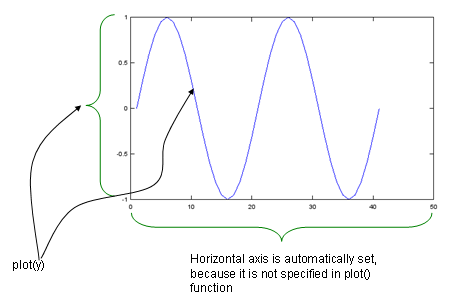< Plot a set of data ((x,y) sequence) >

Ex)

 Input x = -2*pi:1/10*pi:2*pi; y=sin(x); plot(x,y); Output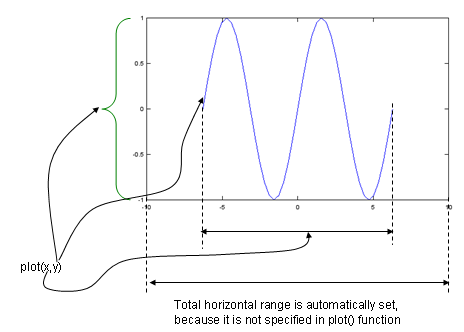< x,y Axis Range >

Case 1 : xlim([xmin xmax]); ylim([ymin ymax]);

Ex)

 Input x = -2*pi:1/10*pi:2*pi; y=sin(x); plot(x,y); xlim([-8 8]); ylim([-1.5 1.5]); Output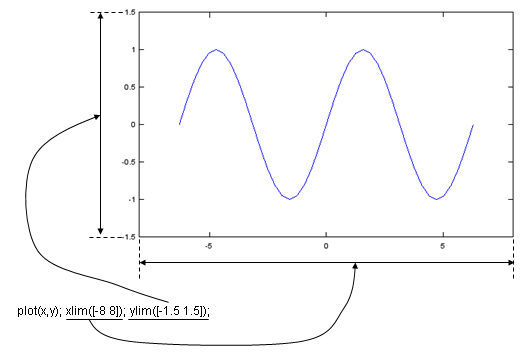Case 2 : axis([xmin xmax ymin ymax]);

Ex)

 Input x = -2*pi:1/10*pi:2*pi; y=sin(x); plot(x,y); axis([-8 8 -1.5 1.5]); Output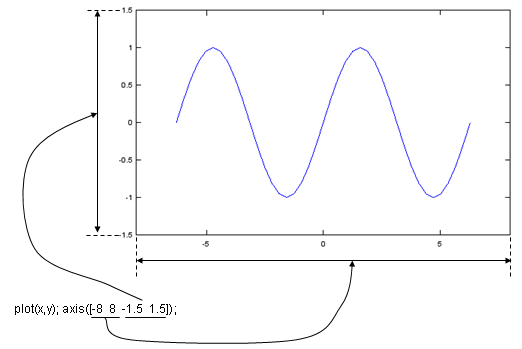< Graph Title, Axis Label >

Case 1 : title('text');

xlabel('text');

ylabel('text');

Ex)

 Input x = -2*pi:1/10*pi:2*pi; y=sin(x); plot(x,y); xlim([-8 8]); ylim([-1.5 1.5]);title('y=sin(x)'); xlabel('x');ylabel('sin(x)'); Output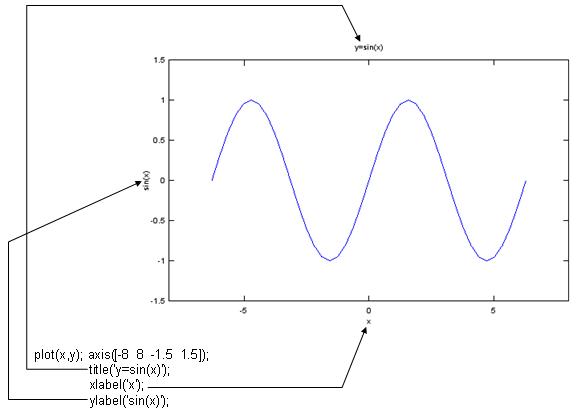< Graph Format and Color >

Case 1 : plot(x,y,'format');

-'  : solid line.

.'  : dotted line.

--' : dashed line

^'  : triangular mark

+'  : + mark

*'  : * mark

o'  : o (circle) mark

x'  : x mark

Ex)

 Input x = -2*pi:1/10*pi:2*pi; y=sin(x); plot(x,y,'r--'); xlim([-8 8]); ylim([-1.5 1.5]);title('y=sin(x)'); xlabel('x');ylabel('sin(x)'); Output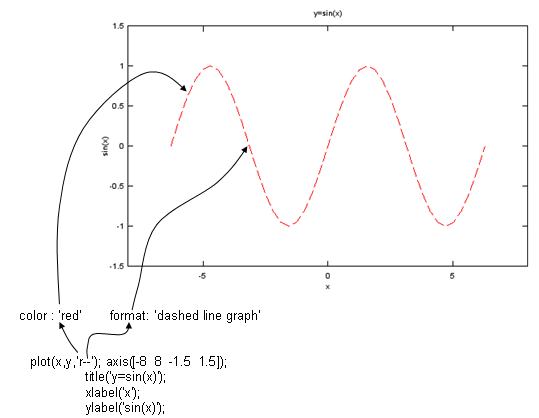Ex)

 Input x = -2*pi:1/10*pi:2*pi; y=sin(x); plot(x,y,'ro'); xlim([-8 8]); ylim([-1.5 1.5]);title('y=sin(x)'); xlabel('x');ylabel('sin(x)'); Output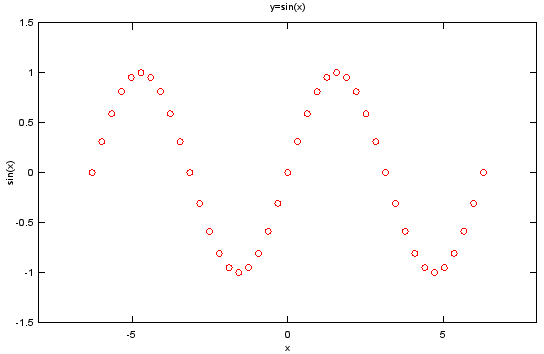Ex)

 Input x = -2*pi:1/10*pi:2*pi; y=sin(x); plot(x,y,'ro-'); xlim([-8 8]); ylim([-1.5 1.5]);title('y=sin(x)'); xlabel('x');ylabel('sin(x)'); Output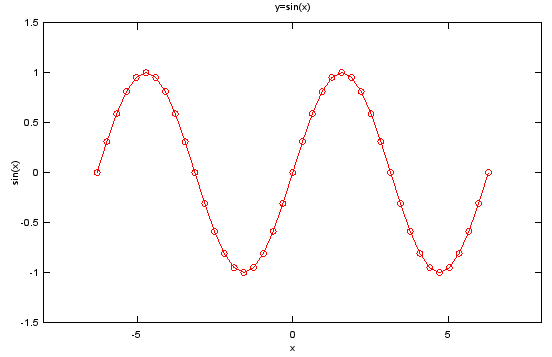< Graph with Filled Marker - MarkerFaceColor>

Case 1 : MarkerFaceColor,[r g b]  // r,g,b value is range between 0 and 1.

Ex)

 Input x = -2*pi:1/10*pi:2*pi; y=sin(x); plot(x,y,'ro','MarkerFaceColor',[0 0 1]); axis([-8 8 -1.5 1.5]); title('y=sin(x)'); xlabel('x');ylabel('sin(x)'); Output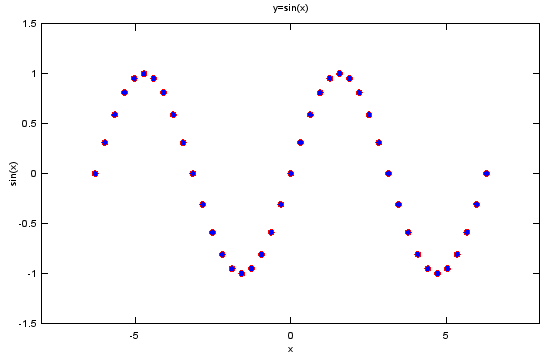Case 1 : MarkerSize,Number

Ex)

 Input x = -2*pi:1/10*pi:2*pi; y=sin(x); plot(x,y,'bo','MarkerFaceColor',[0 0 1],'MarkerSize',4); axis([-8 8 -1.5 1.5]); title('y=sin(x)'); xlabel('x');ylabel('sin(x)'); Output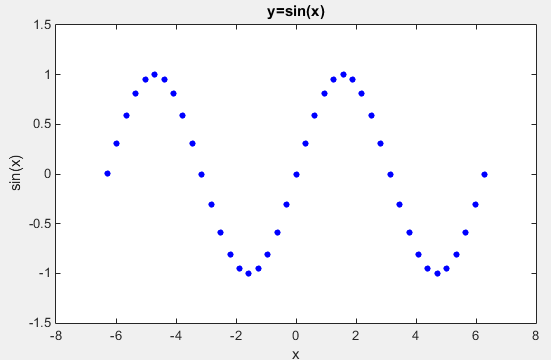< Aspect Ratio >

Case 1 : daspect([xratio yratio])

Ex)

 Input t=0:2*pi/40:2*pi; e1 = exp(t*j); plot(real(e1),imag(e1),'ro','MarkerFaceColor',[1 0 0]); axis([-1.2 1.2 -1.2 1.2]);daspect([1 1]); Output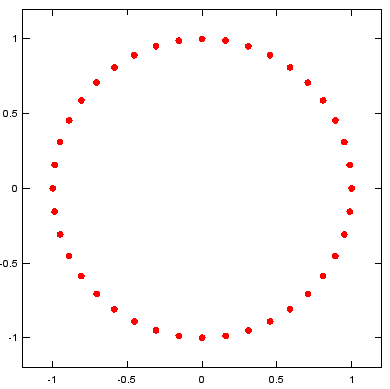< LineWidth >

Ex)

 Input x = [-1 1 1 -1 1 -1]; stairs(x,'LineWidth',2); ylim([-1.5 1.5]); Output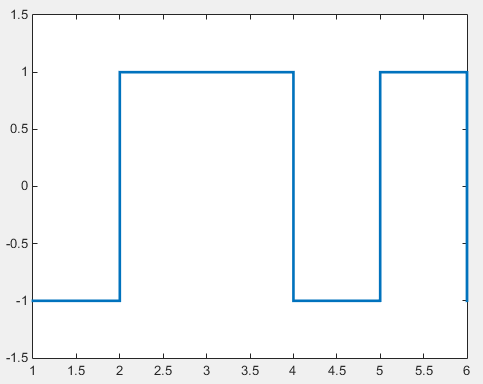< Multiple Plots in a single graph area using plot >

Case 1 : plot(x1,y1,'format1',x2,y2,'format2',...)

Ex)

 Input x = -2*pi:1/10*pi:2*pi; y1=sin(x); y2=0.1 .* x .^2; plot(x,y1,'r-',x,y2,'b-');axis([-8 8 -1.5 1.5]); Output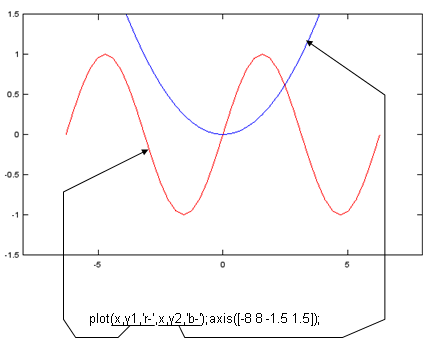< Multiple Plots in a single graph area using hold on >

Ex)

 Input x = -2*pi:1/10*pi:2*pi; y1=sin(x); y2=cos(x); y3=0.1.*x.^2; y4=sin(x).*cos(x); plot(x,y1,'r-');axis([-8 8 -1.5 1.5]);hold on; plot(x,y2,'g-');axis([-8 8 -1.5 1.5]);hold on; plot(x,y3,'b-');axis([-8 8 -1.5 1.5]);hold on; plot(x,y4,'m-');axis([-8 8 -1.5 1.5]); Output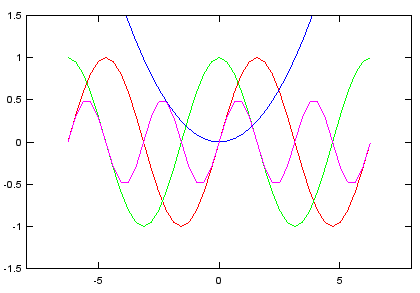< Multiple Plots in a single graph area using subplot >

Case 1 : Subplot(M, N, 1); plot()

Subplot(M, N, 2); plot()

Subplot(M, N, 3); plot()

...

Subplot(M, N, M x N); plot()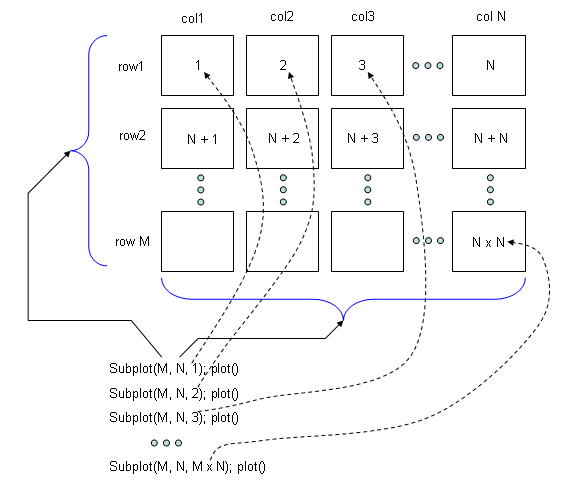Ex)

 Input x = -2*pi:1/10*pi:2*pi; y1=sin(x); y2=cos(x); y3=0.1.*x.^2; y4=sin(x).*cos(x); subplot(2,2,1); plot(x,y1,'r-');axis([-8 8 -1.5 1.5]); subplot(2,2,2); plot(x,y2,'g-');axis([-8 8 -1.5 1.5]); subplot(2,2,3); plot(x,y3,'b-');axis([-8 8 -1.5 1.5]); subplot(2,2,4); plot(x,y4,'m-');axis([-8 8 -1.5 1.5]); Output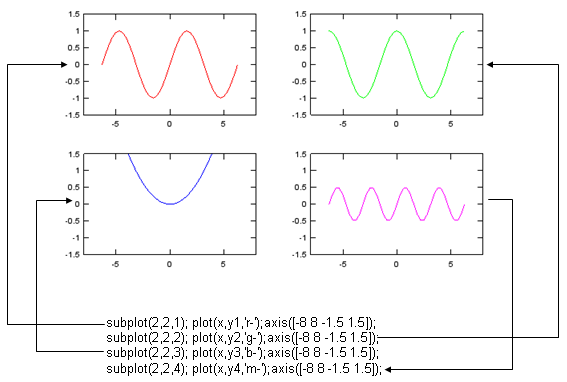< Placing Legend >

Case 1 : legend('string1','string2',...)

Ex)

 Input x = -2*pi:1/10*pi:2*pi; y1=sin(x); y2=0.1 .* x .^2; plot(x,y1,'r-',x,y2,'b-');axis([-8 8 -1.5 1.5]); legend('y1=sin(x)','y2=0.1 .* x .^2'); Output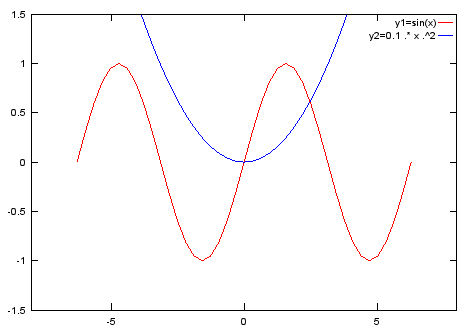< Placing a Text on a plot >

Case 1 : Simple Text

text(x,y,'string')

Ex 01 >

text(-0.5,0.5,'text);

Case 2 : Text with Font Specification

text(x,y,'string', 'FontSize',n,'fontweight','specifier','color','specifier')

Ex 01 >

text(-0.5,0.5,'x(n)','FontSize',16,'fontweight','bold','color','red');

Case 3 : Text with Special Characters

Ex 01 >

text(-1.0,0.7,'\Sigma_{n=0}^{N} x(n) e^{-j (2\pi k n)/N}','FontSize',16,'fontweight','bold');

Case 4 : Text with sprintf()

var = sprintf(...)

text(x,y,var)

Ex 01 >

n = 3;

tStr = sprintf('k = %d',n);

text(-0.5,0.3,tStr,'FontSize',16,'fontweight','bold','color','red');

< Tick/Ticklabel - Turning On/Off >

Case 1 : By default, Tickmark and Ticklabel is set to be ON. You can turn OFF them with set().

set(gca,'xticklabel',[]);

set(gca,'yticklabel',[]);

set(gca,'xtick',[]);

set(gca,'ytick',[]);

Ex)

 Input x = -2:0.1:2; plot(x,x .^ 2); set(gca,'xticklabel',[]);set(gca,'yticklabel',[]); set(gca,'xtick',[]);set(gca,'ytick',[]); Output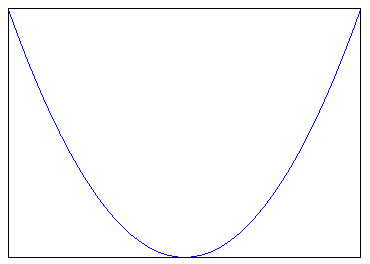< Tick/Ticklabel - User Defined Tick >

Case 1 : set(gca,'xtick',[-2 -1.5 -1 0 1 1.5 2]);

set(gca,'ytick',[0 1 2 3 4]);

Ex)

 Input x = -2:0.1:2; plot(x,x .^ 2); set(gca,'xtick',[-2 -1.5 -1 0 1 1.5 2]); set(gca,'ytick',[0 1 2 3 4]); Output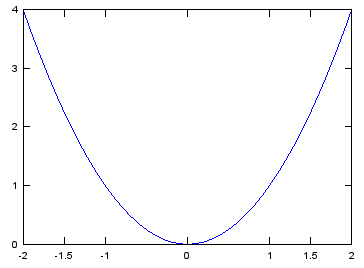< Tick/Ticklabel - User Defined Ticklabel >

Case 1 : set(gca,'xticklabel',{Ticklabel List});

Ex)

 Input t = 0:pi/20:2*pi; plot(t,sin(t)); axis([0 2*pi -1 1]); set(gca,'xtick',[0 pi/2 pi 1.5*pi 2*pi]);set(gca,'ytick',[-1 0 1]); set(gca,'xticklabel',{'0','0.5 pi','pi','1.5 pi','2*pi'}); Output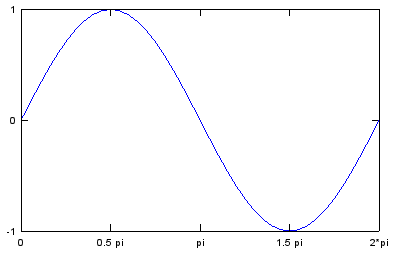< Grid - Turning On/Off >

Case 1 : By default, grid is Off. grid() is toggling grid on/off.

grid()

Ex)

 Input t = 0:pi/20:2*pi; plot(t,sin(t)); axis([0 2*pi -1 1]); set(gca,'xtick',[0 pi/2 pi 1.5*pi 2*pi]);set(gca,'ytick',[-1 -0.5 0 0.5 1]); set(gca,'xticklabel',{'0','0.5 pi','pi','1.5 pi','2*pi'}); grid(); Output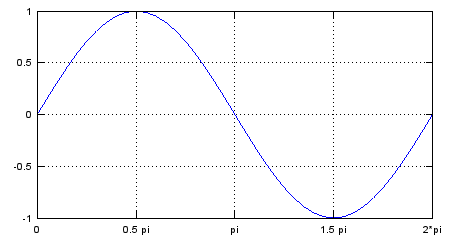< Axis - Turning On/Off >

Case 1 : Turning off the whole axis line, tick mark, tick label

set(gca,'Visible','off')

< Figure window with specified position and size >

Case 1 : in Octave

handle = figure(id,'Position',[x y width height]);

Ex 01 >

hFig = figure(1,'Position',[300 300 800 500]);

Case 2 : in Matlab

handle = figure(id);

...

set(handle,'Position',[x y width height]);

Ex 01 >

hFig = figure(1);

....

set(hFig,'Position',[300 100 800 700]);